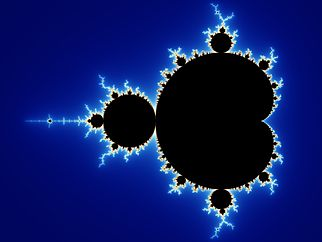## “The Mandelbrot Set” Benoit MandelbrotThe Mandelbrot Set by Benoit Mandelbrot is a fractal that employs the simple equation “z = z *z + c”.  This equation basically controls a feedback loop.  “The equation is calculated dozens, or even millions of times for each pixel. Each time through the loop, the result of one calculation is used as the input […]